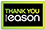Free Delivery  - No Minimum Spend - This week only!  - Last Orders midnight 19th

# Splitting theorems for certain equivariant spectra

PAPERBACK
Category: Mathematics
Let $G$ be a compact Lie group, $\Pi$ be a normal subgroup of $G$, $\mathcal G=G[LAMBDA]Pi$, $X$ be a $\mathcal G$-space and $Y$ be a $G$-space. There are a number of results in the literature giving a direct sum decomposition of the group $[\Sigma^\infty X,\Sigma^\infty Y]_G$ of equivariant stable homotopy classes of maps from $X$ to $Y$. Here, these results are extended to a decomposition of the group $[B,C]_G$ of equivariant stable homotopy classes of maps from an arbitrary finite $\mathcal G$-CW sptrum $B$ to any $G$-spectrum $C$ carrying a geometric splitting (a new type of structure introduced here). Any naive $G$-spectrum, and any spectrum derived from such by a change of universe functor, carries a geometric splitting.Our decomposition of $[B,C]_G$ is a consequence of the fact that, if $C$ is geometrically split and $(\mathfrak F',\mathfrak F)$ is any reasonable pair of families of subgroups of $G$, then there is a splitting of the cofibre sequence $(E\mathfrak F_+\wedge C)^\Pi \rarrow (E\mathfrak F'_+\wedge C)^\Pi \rarrow (E(\mathfrak F',\mathfrak F)\wedge C)^\Pi$ constructed from the universal spaces for the families. Both the decomposition of the group $[B,C]_G$ and the splitting of the cofibre sequence are proven here not just for complete $G$-universes, but for arbitrary $G$-universes.Various technical results about incomplete $G$-universes that should be of independent interest are also included in this paper. These include versions of the Adams and Wirthmuller isomorphisms for incomplete universes. Also included is a vanishing theorem for the fixed-point spectrum $(E(\mathfrak F',\mathfrak F)\wedge C)^\Pi$ which gives computational force to the intuition that what really matters about a $G$-universe $U$ is which orbits $G/H$ embed as $G$-spaces in $U$.
€76.30228 Reward Points
Currently out of stock
Delivery 5-7 Days

Any purchases for more than €10 are eligible for free delivery anywhere in the UK or Ireland!

Let $G$ be a compact Lie group, $\Pi$ be a normal subgroup of $G$, $\mathcal G=G[LAMBDA]Pi$, $X$ be a $\mathcal G$-space and $Y$ be a $G$-space. There are a number of results in the literature giving a direct sum decomposition of the group $[\Sigma^\infty X,\Sigma^\infty Y]_G$ of equivariant stable homotopy classes of maps from $X$ to $Y$. Here, these results are extended to a decomposition of the group $[B,C]_G$ of equivariant stable homotopy classes of maps from an arbitrary finite $\mathcal G$-CW sptrum $B$ to any $G$-spectrum $C$ carrying a geometric splitting (a new type of structure introduced here). Any naive $G$-spectrum, and any spectrum derived from such by a change of universe functor, carries a geometric splitting.Our decomposition of $[B,C]_G$ is a consequence of the fact that, if $C$ is geometrically split and $(\mathfrak F',\mathfrak F)$ is any reasonable pair of families of subgroups of $G$, then there is a splitting of the cofibre sequence $(E\mathfrak F_+\wedge C)^\Pi \rarrow (E\mathfrak F'_+\wedge C)^\Pi \rarrow (E(\mathfrak F',\mathfrak F)\wedge C)^\Pi$ constructed from the universal spaces for the families. Both the decomposition of the group $[B,C]_G$ and the splitting of the cofibre sequence are proven here not just for complete $G$-universes, but for arbitrary $G$-universes.Various technical results about incomplete $G$-universes that should be of independent interest are also included in this paper. These include versions of the Adams and Wirthmuller isomorphisms for incomplete universes. Also included is a vanishing theorem for the fixed-point spectrum $(E(\mathfrak F',\mathfrak F)\wedge C)^\Pi$ which gives computational force to the intuition that what really matters about a $G$-universe $U$ is which orbits $G/H$ embed as $G$-spaces in $U$.
Currently out of stock
Delivery 5-7 Days228 Reward Points

Any purchases for more than €10 are eligible for free delivery anywhere in the UK or Ireland!

€76.30
Currently out of stock
Delivery 5-7 Days228 Reward Points

Any purchases for more than €10 are eligible for free delivery anywhere in the UK or Ireland!

### Product Description

Let $G$ be a compact Lie group, $\Pi$ be a normal subgroup of $G$, $\mathcal G=G[LAMBDA]Pi$, $X$ be a $\mathcal G$-space and $Y$ be a $G$-space. There are a number of results in the literature giving a direct sum decomposition of the group $[\Sigma^\infty X,\Sigma^\infty Y]_G$ of equivariant stable homotopy classes of maps from $X$ to $Y$. Here, these results are extended to a decomposition of the group $[B,C]_G$ of equivariant stable homotopy classes of maps from an arbitrary finite $\mathcal G$-CW sptrum $B$ to any $G$-spectrum $C$ carrying a geometric splitting (a new type of structure introduced here). Any naive $G$-spectrum, and any spectrum derived from such by a change of universe functor, carries a geometric splitting.Our decomposition of $[B,C]_G$ is a consequence of the fact that, if $C$ is geometrically split and $(\mathfrak F',\mathfrak F)$ is any reasonable pair of families of subgroups of $G$, then there is a splitting of the cofibre sequence $(E\mathfrak F_+\wedge C)^\Pi \rarrow (E\mathfrak F'_+\wedge C)^\Pi \rarrow (E(\mathfrak F',\mathfrak F)\wedge C)^\Pi$ constructed from the universal spaces for the families. Both the decomposition of the group $[B,C]_G$ and the splitting of the cofibre sequence are proven here not just for complete $G$-universes, but for arbitrary $G$-universes.Various technical results about incomplete $G$-universes that should be of independent interest are also included in this paper. These include versions of the Adams and Wirthmuller isomorphisms for incomplete universes. Also included is a vanishing theorem for the fixed-point spectrum $(E(\mathfrak F',\mathfrak F)\wedge C)^\Pi$ which gives computational force to the intuition that what really matters about a $G$-universe $U$ is which orbits $G/H$ embed as $G$-spaces in $U$.

### Product Details

#### Splitting theorems for certain equivariant spectra

ISBN9780821820469

FormatPAPERBACK

Publisher (30 March. 2000)

No. of Pages89

Weight201

Language English (United States)

Dimensions 255 x 178 x 7